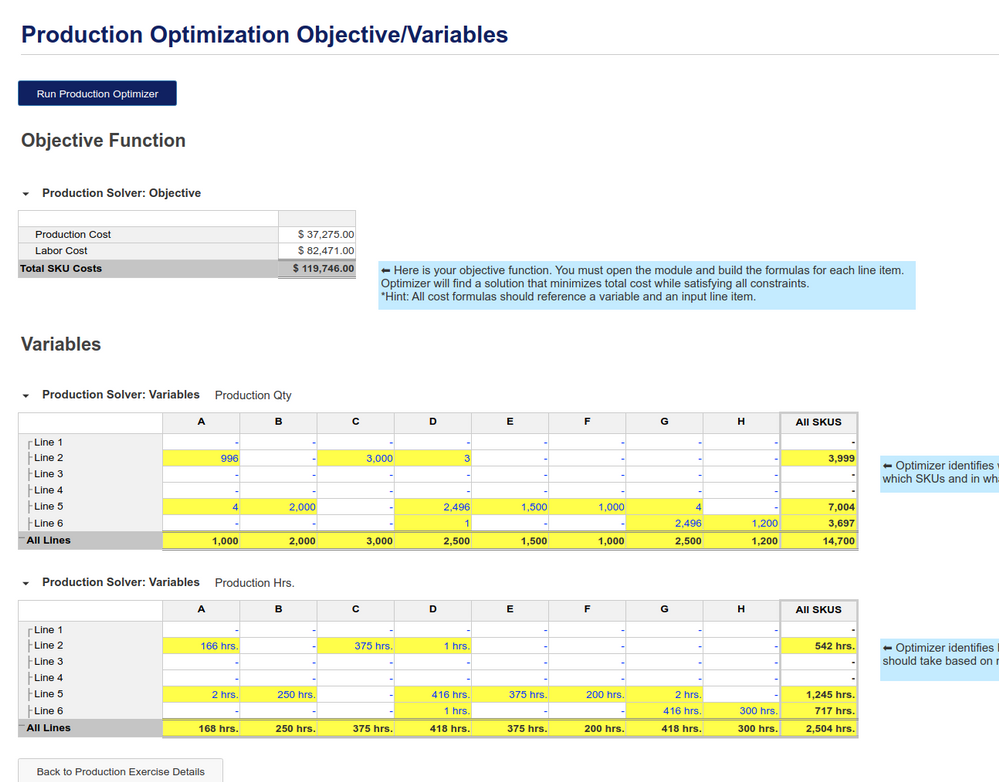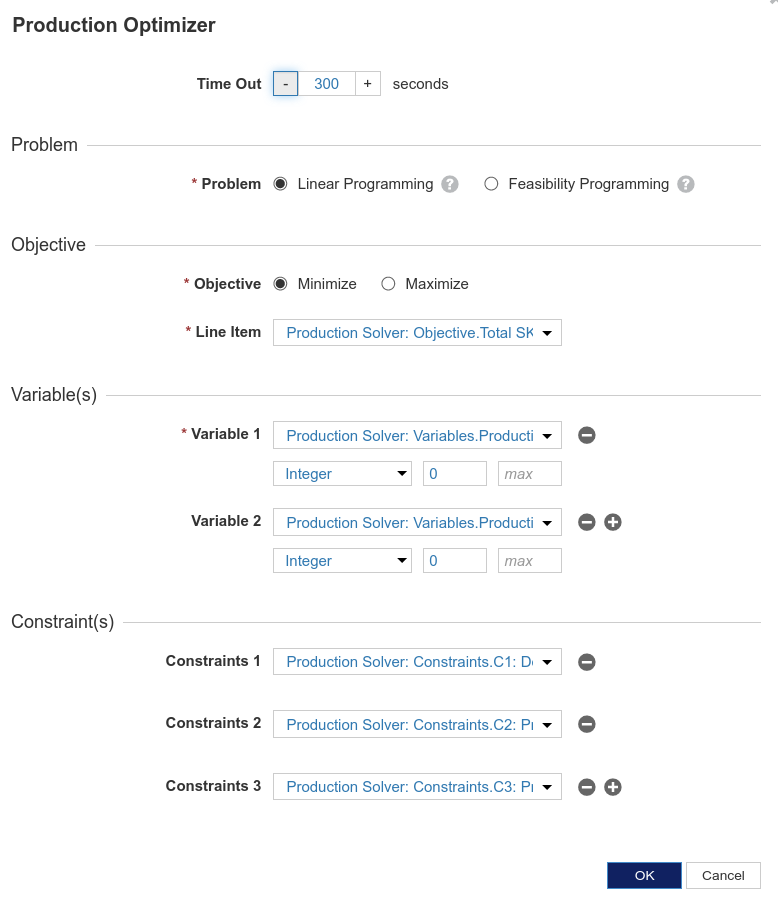# Optimizer Exercises - Production OptimizationHello,

I have just done the Optimizer exercises Optimizer: Exercise Walkthrough created by @mark_godfrey , and have an error in the Production Optimization Exercises example. While its possible I messed with the base data, I'm getting exactly the same answer as a colleague. I compared my answer to Optimizer: Use Case Examples  at minute 10:20. The video shows no small allocations to lines of 1 or 2 SKUs.

The answer I get is different as seen in the "Optimizer: Exercise Walkthrough" video.

I have a demo coming up, so this is a 2 part question.

After running the optimization, I get an allocation of 1,3, or 4 products produced on 1 machine and the balance 2000+ produced on another machine?  I understand optimizer coming to that conclusion, but in reality i think it is an unlikely situation.

My 2 questions are:

1) Does anyone know how I got this solution? (Calcs & Pic Below).

2) How would one go about ensuring that there would be a "Minimum Viable Quantity" for a line to produce to avoid small allocations to lines? - would it be additional constraints? if so what would that look like.

Here are the pictures and formulasCONSTRAINTS

C1 DEMAND: 'Production Solver: InputData'.Demand = 'Production Solver: Variables'.Production Qty
C2 PROD LINE QUANTITY: 'Production Solver: Variables'.'Production Hrs.' <= 'Production Solver: InputData'.Capacity Hrs
C3 PROD. QTY-HRS LINK: 'Production Solver: Variables'.Production Qty - 'Production Solver: Variables'.'Production Hrs.' * 'Production Solver: InputData'.'Run Rate (units/hr)' = 0

OBJECTIVES

PRODUCTION COST: 'Production Solver: Variables'.Production Qty * 'Production Solver: InputData'.Cost Per Piece
LABOUR COST: 'Production Solver: InputData'.Cost Per Labor Hr * 'Production Solver: Variables'.'Production Hrs.'

TOTAL SKU COSTS: Production Cost + Labor CostAny ideas would be most welcome, thanks in advance!

Carrick

Tagged: